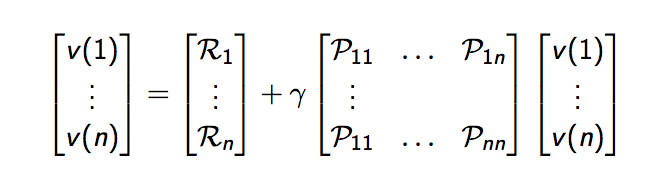When solving reinforcement learning problems, there has to be a way to actually represent states in the environment.

A Markov State is a bunch of data that not only contains information about the current state of the environment, but all useful information from the past. In short, when making decisions based on a Markov State, it doesn't matter what happened, say, three turns ago. Anything that might have changed as a result of past actions will be encoded in the Markov State.

But that only tells about one state of the environment. On the other hand, a Markov Process contains a set of all possible states, and transition probabilities for each one. For example, someone in a sleeping state may have a 70% chance to continue sleeping, and a 30% chance to wake up.

Building on this, a Markov Reward Process contains all the same information, but also has rewards associated with each state. Reward is simply an arbitrary number that determines how good it is to be in a certain state, or take an action from a state. For example, being in the sleeping state may have a reward of 3 as you are well rested, while waking up has a reward of 0. However, reading a book once you wake up may give a reward of 10.

This leads into the concept of reward vs return. Rewards are immediate for each state. However, the return of a state is the total possible reward from that state onwards. There is often a discount associated with future rewards, to account for possible variation and inconsistencies, as well as prevent infinite returns.

The value function of a state indicates its return, which is composed of the state's immediate reward, plus the discounted reward of the future state. In the case of multiple possible states, the future rewards are simply weighted by their respective probabilities.

The Bellman Equation is a simple way of calculating the value of each state. For each state,

``````v = R + γPv
``````With γ being the discount factor (0 <= γ <= 1) and P being the transition probabilities from each state to the next. The Bellman Equation builds on itself, and can be computed iteratively by using the last set of values to compute the latest set.

Finally, a Markov Decision Process puts all the pieces together. It contains everything a Markov Reward Process does, along with a set of actions possible from each state.

This allows a policy, a mapping of states to actions, to be developed. A policy is simply a strategy of which action to take from each state.

By following a policy to determine the next state, bellman equations can be calculated for any number of policies. This brings us to the state-value function, or the expected return for each state, following policy π. There's also an action-value function, which contains expected returns for taking a certain action from a state, following policy π.

The end goal is to find the maximum action-value function over all policies. Once this is known, we can simply always take the action with the highest potential reward, and the agent will theoretically maximize on rewards.

Note: this is a continuation from a previous post, with information taken from David Silver's RL Course.